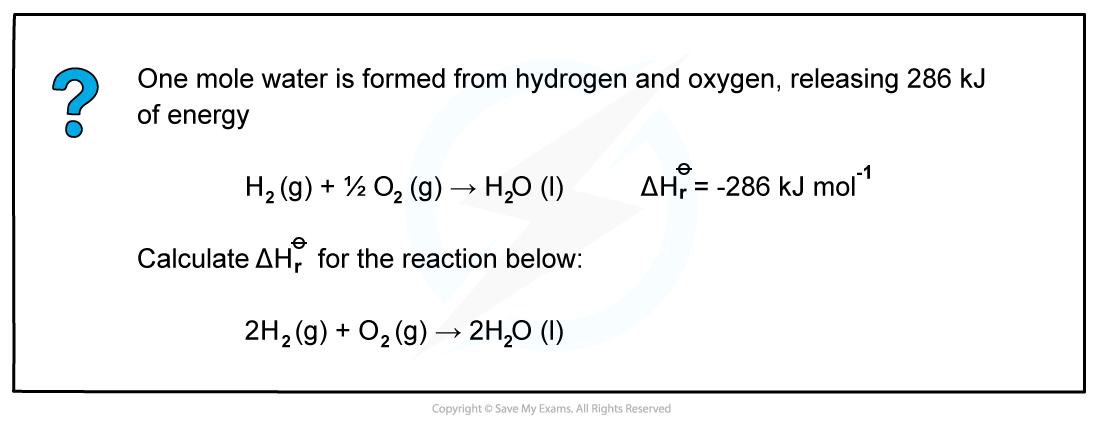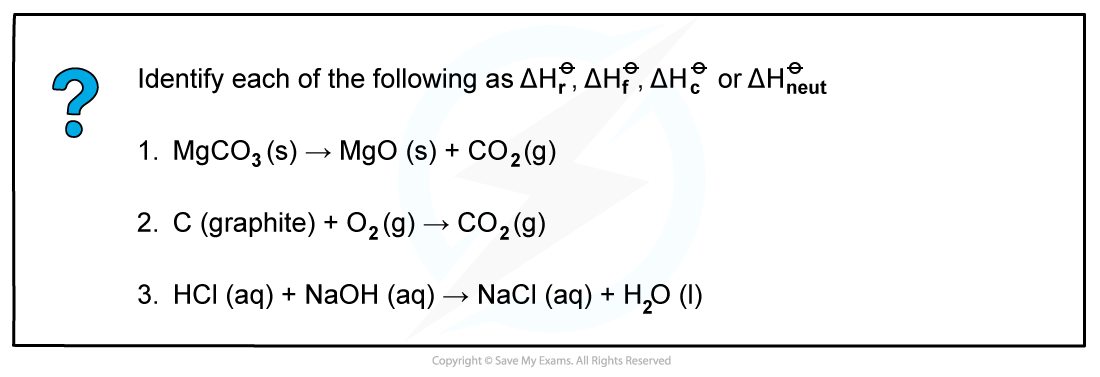# CIE A Level Chemistry复习笔记1.5.3 Standard Enthalpy Change

### Enthalpy Changes at Standard Conditions

• To fairly compare the changes in enthalpy between reactions, all reactions should be carried out under standard conditions
• These standard conditions are:
• A pressure of 101 kPa
• A temperature of 298 K (25 oC)
• Each substance involved in the reaction is in its normal physical state (solid, gas or liquid)
• To show that a reaction has been carried out under standard conditions, the symbol Ꝋ is used
• ΔH = the standard enthalpy change
• These are a number of key definitions for common language relating to enthalpy change that all chemists need to know

Enthalpy definitions table#### Worked example: Calculating the enthalpy change of reaction of waterSince two moles of water molecules are formed in the question above, the energy released is simply:

ΔHr = 2 mol x (-286 kJ mol-1)= -572 kJ mol-1

#### Worked example: Calculating the enthalpy change of formationSince two moles of Fe2O3 (s) are formed the total change in enthalpy for the reaction above is:

ΔHfꝊ =  2 x ( -824.2 kJ mol-1)= - 1648 kJ

#### Worked example: Calculating enthalpy changesAnswer 2: ΔHr as one mole of CO2 is formed from its elements in standard state and ΔHc as one mole of carbon is burnt in oxygen

Answer 3: ΔHneut as one mole of water is formed from the reaction of an acid and alkali

#### Exam Tip

The ΔHf of an element in its standard state is zero.For example, ΔHfof O2(g) is 0 kJ mol-1